Home Practice
For learners and parents For teachers and schools
Textbooks
Full catalogue
Pricing SupportLog in

We think you are located in United States. Is this correct?

# Properties of 3D objects

## 25.3 Properties of 3D objects

There are certain words that we need to use when we describe the properties of 3D objects, such as face, base, edge and vertex.

We know that a cube has six faces, and each face is a square. All the squares are congruent (exactly the same size).

face
the flat surface of a 3D object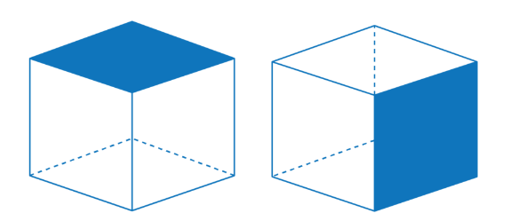The faces of objects meet each other at an edge. A cube has twelve edges: four edges around the top face of the cube, four edges around the side faces, and four edges around the base of the cube.

edge
the line segment along which two faces of a 3D object join together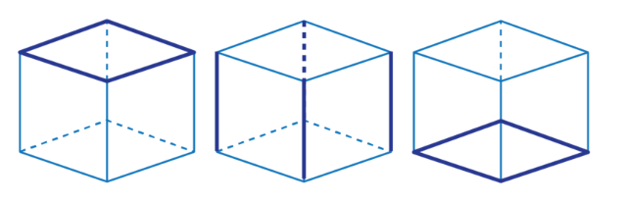The edges of a 3D object meet each other at a vertex. A cube has eight vertices: four at the top of the cube and four at the base of the cube.

vertex
the corner or point where edges of a 3D object meet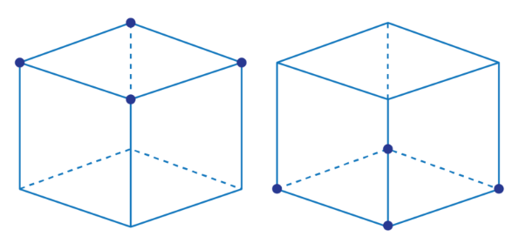# Test yourself now

High marks in science are the key to your success and future plans. Test yourself and learn more on Siyavula Practice.

Exercise 25.1

This shape is a triangular prism.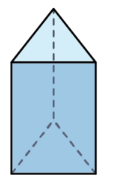1. Count the number of faces.
2. How many edges does it have?
3. How many vertices does it have?
1. $$5$$ faces
2. $$9$$ edges
3. $$6$$ vertices

This shape is a pentagonal prism.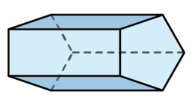1. Count the number of faces.
2. How many edges does it have?
3. How many vertices does it have?
1. $$7$$ faces
2. $$15$$ edges
3. $$10$$ vertices

This shape is called a cuboid or rectangular prism.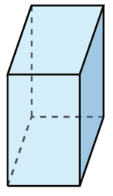Count the number of faces, edges and vertices.

$$6$$ faces, $$12$$ edges and $$8$$ vertices

This shape is a hexagonal prism.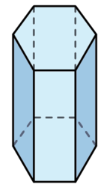Count the number of faces, edges and vertices.

$$8$$ faces, $$18$$ edges and $$12$$ vertices